Courses

# Test: Simplifying Expression

## 15 Questions MCQ Test Quantitative Aptitude for GMAT | Test: Simplifying Expression

Description
This mock test of Test: Simplifying Expression for GMAT helps you for every GMAT entrance exam. This contains 15 Multiple Choice Questions for GMAT Test: Simplifying Expression (mcq) to study with solutions a complete question bank. The solved questions answers in this Test: Simplifying Expression quiz give you a good mix of easy questions and tough questions. GMAT students definitely take this Test: Simplifying Expression exercise for a better result in the exam. You can find other Test: Simplifying Expression extra questions, long questions & short questions for GMAT on EduRev as well by searching above.
QUESTION: 1

### What is the value of the expression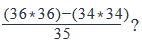Solution: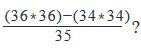= [(1296)-(1156)]/35

= 140/35

= 4

QUESTION: 2

### What is the value of 9986 x 9986?

Solution:

9986*9986=squre of (10000-14)
=100000000-280000+196
=99720196

QUESTION: 3

### Solve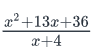when x =1/4 ?

Solution: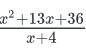where  x =1/4

= [ (1/4)+ 13(1/4) + 36 ] / (1/4) + 4

= [ 1/16 + 13/4 + 36 ] / 17/4

= [ ( 1 + 52 + 576) /16 ] x 4/16

= 629/16 x 4/17

= 37/4

QUESTION: 4

What is the highest integral value of 'k' for which the quadratic equation x2 - 6x + k = 0 will have two real and distinct roots?

Solution:

Step 1: Nature of Roots of Quadratic Equation Theory

D is called the Discriminant of a quadratic equation.
D = b2 - 4ac for quadratic equations of the form ax2 + bx + c = 0.
If D > 0, roots of such quadratic equations are Real and Distinct
If D = 0, roots of such quadratic equations are Real and Equal
If D < 0, roots of such quadratic equations are Imaginary. i.e., such quadratic equations will NOT have real roots.
In this GMAT sample question in algebra, we have to determine the largest integer value that ‘k’ can take such that the discriminant of the quadratic equation is positive.

Step 2: Compute maximum value of k that will make the discriminant of the quadratic equation positive

The given quadratic equation is x2 - 6x + k = 0.
In this equation, a = 1, b = -6 and c = k
The value of the discriminant, D = 62 - 4 * 1 * k
We have compute values of 'k' for which D > 0.
i.e., 36 – 4k > 0
Or 36 > 4k or k < 9.

The question is "What is the highest integer value that k can take?"
If k < 9, the highest integer value that k can take is 8.

QUESTION: 5

If a and b are integers such that a > b > 0, what is the minimum value of  (a2 + b2) (a+b) (a-b) + 2b4?

Solution:

Solution : (a2+b2)(a+b)(a-b) + 2b4

= (a2+b2)(a2 - b2) + 2b4

= (a4 - b4) + 2b4

since a> b > 0

set is a =2 b = 1

value = 15+2 = 17

QUESTION: 6

What is the value of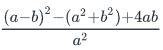where a ≠0?

(i)  a = 8

(ii) b = 16a

Solution:
QUESTION: 7

If (a + b)2 = x and (a – b)2 = y, then what is the value of 2ab in terms of x and y?

Solution:

Step 1: Question statement and Inferences

I:

(a + b)2 = x

a2 + b2 + 2ab = x

Similarly,

II:

(a – b)2 = y

a2 + b2 – 2ab = y

Step 2:Finding required values

If we subtract y from x, we have

x – y = (a2 + b2 + 2ab) – (a2 + b2 -2ab) = 4ab

We have eliminated a2 and b2. We are very close to the answer.

Step 3: Calculating the final answer

The question requires us to find the expression for 2ab. We have already obtained the expression for 4ab.

Lets divide both sides by 2,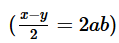QUESTION: 8

Find the value of the expression: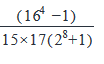Solution:

Step 1: Question statement and InferencesThis question is based on the concept that n2 – 1 = (n – 1)(n + 1)

Step 2 & 3: Finding required values and calculating the final answer

Apply the n2 – 1 concept to the numerator: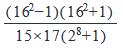Apply the n2 – 1 concept again to the 162 – 1: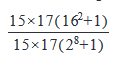Cancel the 15 × 17 from top and bottom: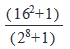Convert 16 to 24 :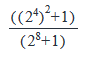Convert (24)2 to 28 :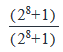QUESTION: 9

If b≠0 and (a/b) +3 = {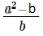}, then 2b =

Solution:

We need to find the value of 2b.

Given equation is: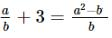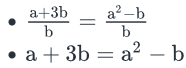(We can safely cancel b from both sides since it is given that b≠0)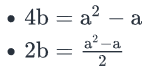QUESTION: 10

If x, y, z are non-zero numbers and x + xy2 = z3, then which of the following is NOT equal to 1?

Solution:

Given equation is

x+xy2 = z3

This can be rewritten as:

x(1+y2) = z …………….. (I)

We need to find the option statement whose value is not equal to 1.

Now let us look at each option.

Option A: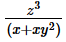This is same as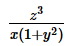…………….. Expression A

From (I), we know that the numerator and denominator of Expression A are equal.

Therefore the value of Expression A is 1.

Option B: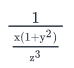This can be rewritten as: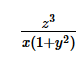We already saw in the previous option that this is equal to 1.

Option C: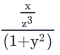This can be rewritten as: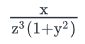From I, we can rewrite this as: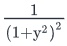………….. Expression C

It is given that y is a non-zero number. Therefore, the value of  (1+y2) is always greater than 1.

So, Expression C cannot be equal to 1.

Option D: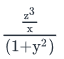This can be rewritten as: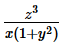From options A and B, we already know that this expression is equal to 1.

Option E: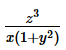We already know that this expression is equal to 1.

QUESTION: 11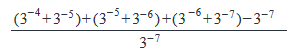is what multiple of 17?

Solution:

Step 1: Question statement and Inferences

Set up the fraction without the brackets so you can simplify it.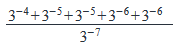Step 2 & 3: Simplifying the fraction and calculating the final answer

Turn the bottom negative exponent into a fraction: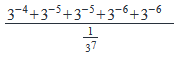Multiply the numerator and denominator with 37:

(3−4+3−5+3−5+3−6+3−6)×37

Distribute the 37 among the other exponents:

(33+32+32+31+31)

Simplify the remaining numbers:

(27+9+9+3+3)

51

51 = 17 * 3

QUESTION: 12

What is the sum of the squares of x2 and y2?

(1) x8 – y8 = 6

(2) x4 – y4 = 2

Solution:

Steps 1 & 2: Understand Question and Draw Inferences

We need to find the sum of the squares of x2 and y2.

Square of x2 = (x2)2 = x4

Square of y2 = (y2)2 = y4

Therefore we need to find the value of the expression x4 + y4

Step 3: Analyze Statement 1

Statement (1) says:

x8 – y8 = 6

We know that:  x8 – y8 = (x4 + y4)(x4 – y4 )

[Formula used:   (a- b2) = (a + b)(a - b)

This means that,(x4 + y4)(x4 – y4 ) = 6 ……………… (I)

However, we don’t know the value of the expression (x4 – y4 )

Therefore statement 1 alone is not sufficient to arrive at a unique answer

Step 4: Analyze Statement 2

Statement (2) says:

(x4 – y4 ) = 2 ……………………(II)

However, this alone doesn’t help us in finding the value of (x4 + y4)

Therefore statement 2 alone is not sufficient to arrive at a unique answer.

Step 5: Analyze Both Statements Together (if needed)

Now let us look at both the statements together.

Using (II) in (I), we get:

(x4 + y4) * 2 = 6

(x4 + y4) = 3

Statement (1) and statement (2) together are sufficient to arrive at a unique answer.

QUESTION: 13

For how many real values of x is this expression equal to zero?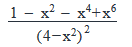Solution:

Step 1: Question statement and Inferences

The fraction is equal to zero when the numerator is equal to zero.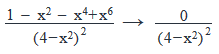Step 2 & 3: Simplifying the fraction and calculating the final answer

For the fraction to equal zero, (x2 + x4 -x6) has to equal 1 to subtract with the 1 on its left: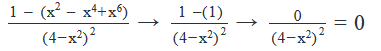The only way that (x2 + x4 -x6) can equal 1 is if x = 1 or –1. There are two possible values of x:  1 and –1.

Note that x cannot equal 2 or –2, making the denominator equal 0. This would result in a non-real number, which is not equal to 0.

QUESTION: 14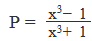, where x ≠ -1 and x ≠ 1

If x is replaced with - 1/x in the expression P, the expression Q is obtained. Which of the following is the correct relationship between P and Q?

Solution:

Step 1: Question statement and Inferences

We have to replace x everywhere in the given expression for P with −1/x

Step 2: Finding required values

Replacing x with −1/x

, we get Q.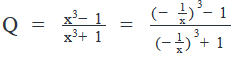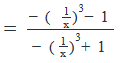Multiplying the numerator and the denominator with -1, we have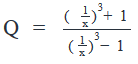Multiplying the numerator and the denominator with x3, we have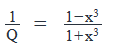Step 3: Calculating the final answer

Notice the similarity between P and Q.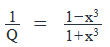Are P and 1/Q  equal? No.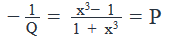In other words, PQ = -1

QUESTION: 15

If y >2, and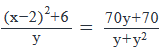which of the following could be the value of 2x + 3?

Solution:

Given equation is:(Let’s call the above equation as I)

We need to find the value of 2x + 3

On the RHS of I, notice that:

1. The numerator: 70y+70=70(y+1)
2. The denominator: y+y2=y(1+y)

Therefore I can written as: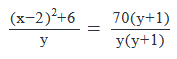Observe that you can cancel the common term   in the numerator and denominator.

Similarly, you can cancel the common term   in the denominator of LHS and the denominator of RHS.

(Note that it is given that y>2 and therefore neither y nor y+1 can be zero. So we can safely cancel these terms out.)

(x−2)2+6=70

•
• (x−2)2=64
•
• (x−2)=±8
•

Case 1:

(x - 2) = 8

• x = 10
• 2x + 3 = 23

Case 2:

(x - 2) = -8

• x = -6
• 2x + 3 = -9

In the given options, -9 is given.